APP Information

 Download Version 13.2.1 (18) Apk Size 5.63 MB App Developer Daan Malware Check TRUSTED Install on Android 4.1.x and up App Package com.locus.scientificcalculator.apk MD5 c604ca1c5a1f5a0ef662738a6eac8e44 Rate 5 Website https://c179d1eae.app-ads-txt.com/app-ads.txt### App Description

```A power calculator that helps solve math formulas with various functions such as permutation and combinations. The scientific calculator also comes with logarithm, exponential, and modulus operations.

With the power calculator, solve various arithmetic problems and math formulas for school or work. Stellar is a great app for any student studying mechanical engineering, physics, or mathematics.

The calculator app includes fractions, complex numbers, advanced statistics, and history and memory registers as well as trigonometry formula functions using Sin Tan Cos.

Scientific Calculator Feature Summary:
- Logarithm and exponential functions
- Permutation and combinations
- Save your values in 9 custom memories
- Copy & paste Clipboard support
- Solve multiple calculations at the same time
- View previous calculations history
- Arithmetic & Trigonometric functions
- Operations for basic mathematics such as addition, subtraction, division, multiplication, and modulus
- Texas Calculator
- Can be used as a School Calculator
- Engineering calculator
- Geometry Calculator

Great for anyone looking for a math Calculator to solve their daily arithmetic problems.
```

### App ChangeLog

• DEL buttons now works perfectly, improved stability, performance improvements, reduced file size.

### More Android Apps to Consider

1.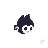Zeki Kah

This calculator is almost exactly what I am looking for, but as of now if you want to change your answer or fix up your mistake, you have to rewrite the whole equation. Which is too bad because I really wanted to use this as the main calculator on my phone

2.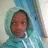Brian Makiwa

Very intuitive easy to use...very akin to what we used in school

3.Rafia Imran

This calculator is very simple to use and saves our time and money too

4.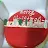Marie Fe Biacolo

It is nice and easy to do a computation like an original calculator i handed...thanks for this apps..

5.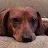J. Sokalski

Very good calculator. Complete functions and works just like a real handheld. Excellent

6.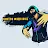Vernon Glinton

Its a great calculator and I am still finding ways to use it still.

7.Milind Chande

Great! As good as a standalone Casio scientific calculator!

8.Nawab Sahib

This calculater is so usefull for me my own calculater was broke then i download this calculater THANKYOU SO MUCH if i do not install it then my numericals will never be completed thank you

9.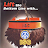ISC CAL

It was refreshing experience as compared to other calculator apps

10.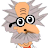Ian Lang

Good device, does the job and easy to work it.